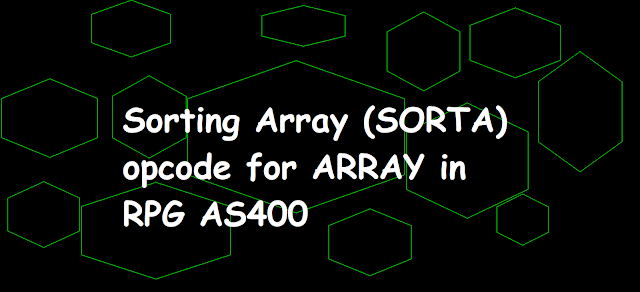Sorting Array (SORTA) opcode for ARRAY in RPG AS400 - AS400 and SQL Tricks

# Sorting Array (SORTA) opcode for ARRAY in RPG AS400

Sorting Array (SORTA) opcode for ARRAY in RPG AS400Sorting Array (SORTA) opcode for ARRAY in RPG AS400

## Coding SORTA opcode in RPG Fixed Format

SORTA opcode is used to sort the array elements in the RPG program. We can use this opcode to sort an Array or a DS array but in this blog, we will discuss sort an Array only.

### Coding SORTA opcode in RPG fixed format to Sort an Array

```     DArray            S              1A   DIM(5)
C                   EVAL      ARRAY(1) = 'X'
C                   EVAL      ARRAY(2) = 'A'
C                   EVAL      ARRAY(3) = 'C'
C                   EVAL      ARRAY(4) = 'B'
C                   EVAL      ARRAY(5) = 'R'
C                   SORTA     ARRAY
C     ARRAY(1)      DSPLY
C     ARRAY(2)      DSPLY
C     ARRAY(3)      DSPLY
C     ARRAY(4)      DSPLY
C     ARRAY(5)      DSPLY
C                   SETON                                        LR
```

### Output

The array has been sorted in Ascending order as can be seen from the below output.

```DSPLY  A
DSPLY  B
DSPLY  C
DSPLY  R
DSPLY  X ```

## Using SORTA opcode to Sort an Array in RPG /Free and Fully Free Format

SORTA operation with operation extender A(Ascending) or D(Descending) can be specified to sort array in ascending or descending order accordingly.

`SORTA(A/D) arrayname `

### Coding SORTA(A/D) opcode in /FREE and Fully free format RPGLE

Let's take an example where we have 3 arrays to be sorted.

• Array1 has the keyword ASCEND so the array will be sorted in Ascending order default
• Array2 has the keyword DESCEND so the array will be sorted in Descending order default
• Array3 has no keyword so the array will be sorted in descending order as we use SORTA opcode with operation extender D.
• RPG Code in /Free format for SORTA (sort an Array) in RPGLE AS400
```     DArray1           S              1A   DIM(5) ASCEND
DArray2           S              1A   DIM(5) DESCEND
DArray3           S              1A   DIM(5)
/Free
ARRAY1(1) = 'X';
ARRAY1(2) = 'A';
ARRAY1(3) = 'C';
ARRAY1(4) = 'B';
ARRAY1(5) = 'R';

SORTA Array1;

DSPLY ARRAY1(1);
DSPLY ARRAY1(2);
DSPLY ARRAY1(3);
DSPLY ARRAY1(4);
DSPLY ARRAY1(5);
ARRAY2(1) = 'X';
ARRAY2(2) = 'A';
ARRAY2(3) = 'C';
ARRAY2(4) = 'B';
ARRAY2(5) = 'R';

SORTA Array2;

DSPLY ARRAY2(1);
DSPLY ARRAY2(2);
DSPLY ARRAY2(3);
DSPLY ARRAY2(4);
DSPLY ARRAY2(5);

ARRAY3(1) = 'X';
ARRAY3(2) = 'A';
ARRAY3(3) = 'C';
ARRAY3(4) = 'B';
ARRAY3(5) = 'R';

SORTA(D) Array3;

DSPLY ARRAY3(1);
DSPLY ARRAY3(2);
DSPLY ARRAY3(3);
DSPLY ARRAY3(4);
DSPLY ARRAY3(5);
*INLR = *ON;
/End-Free
```
RPG Code in Fully Free format for SORTA (sort an Array) in RPGLE AS400
```**FREE
ctl-opt debug(*yes);
ctl-opt Option(*NoDebugio);

dcl-s Array1 char(1) DIM(5) Ascend;
dcl-s Array2 char(1) DIM(5) Descend;
dcl-s Array3 char(1) DIM(5);

ARRAY1(1) = 'X';
ARRAY1(2) = 'A';
ARRAY1(3) = 'C';
ARRAY1(4) = 'B';
ARRAY1(5) = 'R';

SORTA Array1;

DSPLY ARRAY1(1);
DSPLY ARRAY1(2);
DSPLY ARRAY1(3);
DSPLY ARRAY1(4);
DSPLY ARRAY1(5);
ARRAY2(1) = 'X';
ARRAY2(2) = 'A';
ARRAY2(3) = 'C';
ARRAY2(4) = 'B';
ARRAY2(5) = 'R';

SORTA Array2;

DSPLY ARRAY2(1);
DSPLY ARRAY2(2);
DSPLY ARRAY2(3);
DSPLY ARRAY2(4);
DSPLY ARRAY2(5);

ARRAY3(1) = 'X';
ARRAY3(2) = 'A';
ARRAY3(3) = 'C';
ARRAY3(4) = 'B';
ARRAY3(5) = 'R';

SORTA(D) Array3;

DSPLY ARRAY3(1);
DSPLY ARRAY3(2);
DSPLY ARRAY3(3);
DSPLY ARRAY3(4);
DSPLY ARRAY3(5);
*INLR = *ON;
```